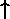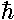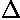## Spin and Spin-Statistics

The Spin-Statistics Theorem concludes wavefunctions must have definite symmetry (even or odd) when the coordinates of identical particles are exchanged. So, for example, all multi-electron wavefunctions must have the property that if the coordinates of any two electrons are exchanged, the resulting function is (-1)× the original function. Electron wavefunctions must be antisymmetric (i.e., "odd under exchange"). On the other hand, all multi-pion wavefunctions must have the property that if the coordinates of any two pions are exchanged, the resulting function is the same as the original function (i.e., "even under exchange"). A particle is called a fermion if its wavefunctions must be odd under exchange. A particle is called a boson if its wavefunctions must be even under exchange.

The Spin-Statistics Theorem concludes that the determining feature as to whether a particle is a boson or a fermion is the spin of the particle. Just as the planet Earth is both spinning on its axis and orbiting the Sun, so electrons are spinning on their axis while orbiting the nucleus in an H-atom. With enough energy the Earth could be made to spin slower or faster [in fact the Earth's spin is slowing over aeons] or orbit with more or less angular momentum. While the electron's orbital angular momentum can change (i.e., various integer values of l are allowed), it always is seen with exactly the same spin-rate. The only possible change that can be made to an electron's spin is to change the direction of the spin orientation: "up" () or "down" (). Thus the spin angular momentum of a fundamental particle is an unchanging characteristic of the particle. The rules of quantum mechanics guarantee that spin angular momentum of a particle must be [s(s+1)]½, where s (hereafter called the "spin of the particle") is either a whole number (0, 1, 2,...) ( so called "integer spin") or half of a odd whole number (1/2, 3/2, 5/2, ...) (so called "half integer spin"). Integer spin particles are always bosons; half integer spin particles are always fermions.

Fermions
Particle
Name
Spin
quarks1/2
electron1/2
proton1/2
neutron1/23/2
He3 nucleus1/2
O17 nucleus5/2
Bosons
photon1
gluon1
graviton2
pion0
H2 nucleus1
He4 nucleus0
B10 nucleus3

On the previous page we made both symmetric and antisymmetric two electron wavefunctions. However our wavefunctions just included the spatial part of the wavefunctions. The spin part of a two electron wavefunction can be:

symmetric:, (+),antisymmetric: (-),

(Notation: the first arrow in a pair refers to electron 1 spin and the second arrow to electron 2 spin.)

Thus in order to made an overall antisymmetric wavefunction we need to pair one of the three symmetric spin wavefunctions with an antisymmetric spatial wavefunction (hence the name: triplet), or pair the single antisymmetric spin wavefunction with a symmetric spatial wavefunction (hence name: singlet)

Thus the ground state of Helium can be written:

1s(r1)1s(r2) (-),

One of the excited states considered on the previous page might be written:

(1s(r1)2p(r2) - 2p(r1)1s(r2))Note that is impossible to create a totally antisymmetric function of three electron spins. Thus the Pauli Exclusion Principle that at most two electrons can be in the same spatial wavefunction. (If two electrons share a spatial wavefunction, they must be in the spin "paired", i.e., singlet, state.)

Thus two electrons can fit in the 1s state, six electrons can fit in the 3 (m=-1,0,1) 2p states, and ten electrons can fit in the 5 3d states.

One is tempted to connect the characteristic of 2 electrons "filling up" an orbital, with the naive idea that "you can't put two things in the same place". However, note that a Uranium atom, with its 92 electrons "filling" the orbitals with n<5, will happily latch on to the negatively charged muon (µ-: a spin 1/2 particle similar to the electron but about 200 times more massive) which can occupy its own 1s orbital. However if three muons are attached to Uranium, the third must go in the 2s state. Any number of negatively charged pions (a psin 0 particle a bit more massive than the muon) can enter the 1s state, even though there are two muons and two electrons in the 1s state. That is to say that the Spin-Statistics Theorem says nothing about the exchange properties of different types of particles, only identical particles.